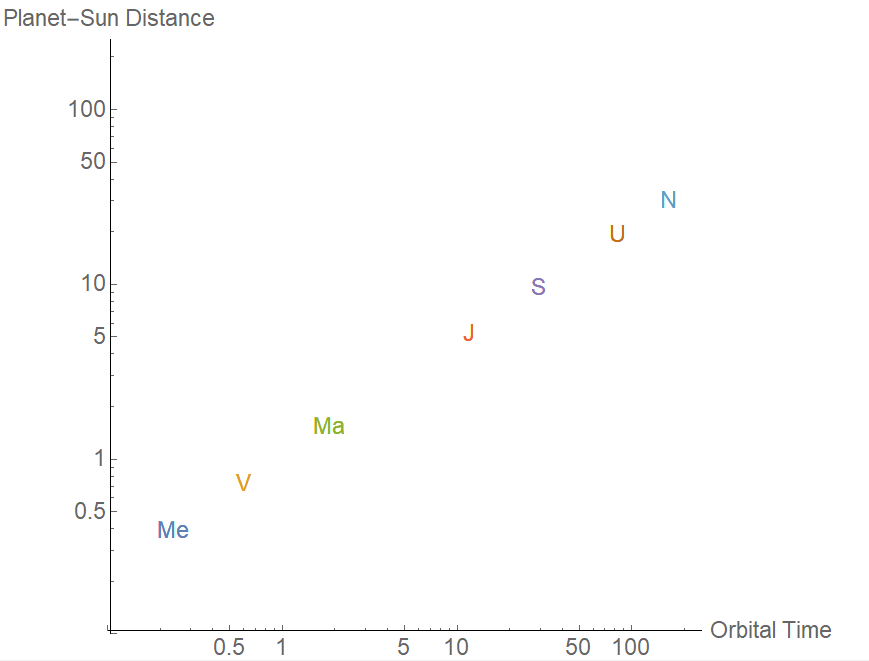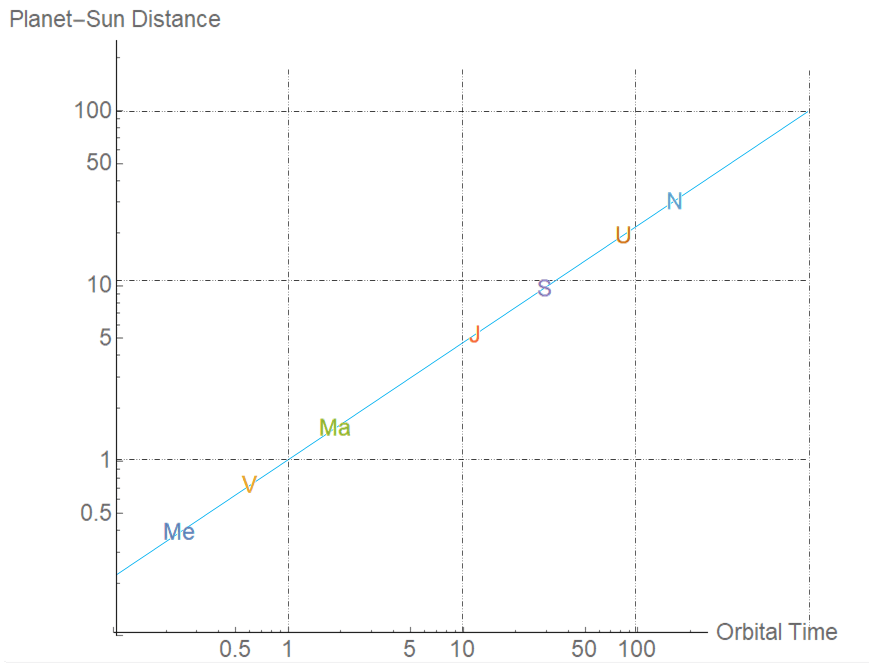# Breakthrough! Do-it-Yourself Astronomy Pays Off: A Law of Nature (and a Bonus)

In recent posts (here and here), I showed you methods that anyone can use for estimating the planets’ distances from the Sun; it just takes high-school trigonometry. And even more recently I showed how, using just algebra, you can easily obtain the planets’ orbital periods from their cycles as we see them from Earth, starting with one solar conjunction and ending at the next.

Much of this work was done by Nicolai Copernicus himself, the most famous of those philosophers who argued for a Sun-centered universe rather than an Earth-centered universe during the millennia before modern science. He had all the ingredients we have, minus knowledge of Uranus and Neptune, and minus the clues we obtain from telescopes, which would have confirmed he was correct.

Copernicus knew, therefore, that although the planetary distances from the Sun and their cycles in the sky (which astrologers [not astronomers] have focused on for centuries) don’t seem to be related, the distances and their orbital times around the Sun are much more closely related. That’s what we saw in the last post.

Let me put these distances and times, relative to the Earth-Sun distance and the Earth year, onto a two-dimensional plot. [Here the labels are for Mercury (Me), Venus (V), Mars (Ma), Jupiter (J), Saturn (S), Uranus (U) and Neptune (N).] The first figure shows the planets out to Saturn (the ones known to Copernicus).Figure 1: The planets’ distances from the Sun (in units of the Earth-Sun distance) versus the time it takes them to complete an orbit (in Earth-years). Shown are the planets known since antiquity: Mercury (Me), Venus (V), Mars (M), Jupiter (J) and Saturn (S).

The second shows them out to Neptune, though it bunches up the inner planets to the point that you can’t really see them well.Figure 2: Same as Figure 1, but now showing Uranus (U) and Neptune (N) as well.

You can see the planets all lie along a curve that steadily bends down and to the right.

Copernicus knew all of the numbers that go into Figure 1, with pretty moderate precision. But there’s something he didn’t recognize, which becomes obvious if we use the right trick. In the last post, we sometimes used a logarithmic axis to look at the distances and the times. Now let’s replot Figure 2 using a logarithmic axis for both the distances and the times.Figure 3: Same as Figure 2, but now with both axes in logarithmic form.

Oh wow. (I’m sure that’s the equivalent of what Kepler said in 1618, when he first painstakingly calculated the equivalent of this plot.)

It looks like a straight line. Is it as straight as it looks?Figure 4: Same as Figure 3, but with a blue line added to show how well a straight line describes the distance/time relationship of the planets, and with a grid added that passes through 1, 10, 100, 1000 Earth-years and distances equal to 1, 10, 100 Earth-Sun distances.

And now we see three truly remarkable things about this graph:

• First, the planet’s distances to the Sun and orbital times lie on a very straight line on a logarithmic plot.
• Second, the slope of the line is 2/3 (2 grid steps up for every 3 steps right) rather than, say, 7.248193 .
• Third, the line goes right through the point (1,1), where the first horizontal and first vertical lines cross.

What do they mean?

### Why Does The Line Pass Through the Point (1,1)?

The line that runs through Figure 4 happens to intersect the point where the distance is the Earth-Sun distance and the orbital time is one Earth-year. Not that there’s a planet there, and in fact there are no planets on any of the grid points in Figure 4. Is this a random, lucky accident? Or might it be telling us something?

Well… hmmm… even before we know why there’s a straight line in Figure 4, this feature’s quite suggestive. Suppose there were another planet whose orbital radius happened to be the same as the Earth-Sun distance (not that I’m saying there is one) and it followed the same rules, whatever they might be, as the other planets (not that I’m saying that it does). Then its orbit around the Sun, according to this graph, would be one familiar year.

Gosh. Could it be… that the Earth… ??

Hey, let’s not jump to conclusions. It’s just circumstantial evidence, not (cough) a proof that the Earth is itself a Sun-orbiting planet, just like the planets we see in the sky. But it’s… thought-provoking.

[Copernicus and Kepler believed the Earth is a Sun-orbiting planet like the others, and Kepler already knew that, with this assumption, Earth’s orbit is a near-circular ellipse, like those of the other planets. So Kepler included the Earth when he found this relationship between orbits and distances. But there wasn’t independent proof, and indeed Kepler’s mentor Tycho Brahe, who did all the observations which Kepler needed for his precise understanding of the planets, died in 1601 still believing the Sun orbits the Earth (because we don’t feel the motion or see the stars shift over the year.) So in this and future posts, we’ll continue with suspended judgment until we can find stronger evidence one way or the other.]

### What the Straight Line With Slope 2/3 Means

The straight line means there is a power-law relationship between orbit distances and orbit times. Let me explain that.

We all learn about straight lines and parabolas in school; I’ve plotted one of each in the left of the figure below, along with a perfect cube and a square root too. What happens to these shapes if we put them on logarithmic axes?Figure 5: Power laws y=xp , shown for p=1/2, 1, 2, 3 in the left plot, become straight lines with slope p when both axes are put into logarithmic form. This is the graphical realization of the statement that log(y) = log(xp) = p log(x) .

They all become straight lines. And the slopes of these lines are 1/2 for the square root, 1 for the straight line, 2 for the parabola and 3 for the cubic. More generally, if y = xp (a “power law”), then on a graph with logarithmic axes this power law will appear as a straight line with slope p. That’s because

• log(y) = log(xp) = p log(x) .

Since the blue line in Figure 4 has slope 2/3, the planet-Sun distances R are related to orbital periods T by the relation R=T2/3. Cubing both sides, we can put it in its more famous form,

• R3=T2

where T is measured in Earth-years, and R counts the number of Earth-Sun distances. This is known as Kepler’s third law of planetary orbits. (It works whenever there’s a central object around which multiple objects orbit, such as the moons of Jupiter.) What deeper causes lie behind this law? We’ll look into that soon.

If you’ve gone through my full set of posts, from the Earth’s shape and spin to the layout of the planets, then you’ve discovered this regularity of nature yourself, starting from scratch. Congratulations! You didn’t need me to tell you Kepler’s third law; I was just your guide. Doesn’t seem so hard, actually; no college education or Einsteinian brilliance necessary.

But if it’s that easy, why didn’t Copernicus immediately figure this out back in the mid-1500s, when he obtained much of the information that goes into Figure 1? We can talk about that in a future post.

### Kepler’s Highly Precise “Law”

Now, I hasten to add that what we’ve really found is Kepler’s law in the approximation that the planet orbits are nearly circular. Kepler himself insisted on precise answers, because he was well aware how acceptance of imprecision had led the Greeks astray, as well as Copernicus. He knew the orbits of the planets weren’t quite circular and were all a little different. He first tried ovals, but they didn’t work. Then he tried ellipses, and found they work perfectly, as long as the Sun is at one focus of the ellipse. But after that, it took him more than ten years to come to the T3=R2 relation. Here’s one reason: if a planet’s orbit is not a circle, then what, exactly, is R? The average planet-Sun distance? The smallest distance? The largest? The square root of the area of the ellipse? None of them work.Each planet’s orbit is an ellipse (with the Sun at one focus.) Which distance should be compared with the orbital times? Is it a, b, c, d, e, or some complex combination of these, such as (c+d)/2 or the square root of ab?

After playing around with the data and the math for many years, he finally found the answer in 1618. To very high precision — no rough estimates here — the formula works spectacularly well as long as you take R to be half the distance across the long diameter (the “semi-major axis”) of the ellipse (drawn as “b” in the figure.)

All this was possible because Kepler’s mentor Tycho Brahe had made extremely precise measurements of the planets’ locations on the sky, and because Kepler knew how to apply what was then advanced mathematics to the problem. The precision of his result, not the mere idea of a Sun-centered universe (which itself was not new), is what set modern astronomy on its path forward to the present. If you really want to understand what Kepler achieved, you will need to go deeper than a simple do-it-yourself approach.

But we have come a long way with these simple methods. Let’s see how much further we can go with them.

### 4 thoughts on “Breakthrough! Do-it-Yourself Astronomy Pays Off: A Law of Nature (and a Bonus)”

1.“What deeper causes lie behind this law? We’ll look into that soon.”
Will this be a future post? I’m curious to know the “deeper causes” behind this law. So far you only stated the observations. Why is there such a relation? Do we know?

•Yes, it will be a future post, as soon as the heat and light over the W boson mass measurement dies down a little…

2.What is “mass” in this context? Can this be explained in the style of this series of articles that you have been publishing? I mean without assuming anything as you do in these artcles.

•It can be explained, but the mystery of what mass is wasn’t solved until the 20th century, and it’s well beyond my current purposes. I do expect to explain it in the book I’m currently drafting.